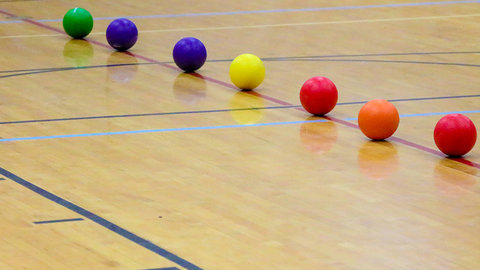# More Than One Infinity Episode 7 of Mind-Bending Math: Riddles and Paradoxes

##### The Great CoursesShow More

## Related videos

Cantor's Infinity of Infinities
Randomly pick a real number between 0 and 1. What is the probability that the number is a fraction, such as 1/4? Would you believe that the probability is zero? Probe this and other mind-bending facts about infinite sets, including the discovery that made Cantor exclaim, "I see it, but…
The Joy of Infinity
Episode 16 of The Joy of Mathematics
What is the meaning of infinity? Are some infinite sets "more" infinite than others? Could there possibly be an infinite number of levels of infinity? This lecture explores some of the strange ideas associated with mathematical infinity.
Infinity Is Not a Number
The paradoxes associated with infinity are... infinite! Begin with strategies for fitting ever more visitors into a hotel that has an infinite number of rooms, but where every room is already occupied. Also sample a selection of supertasks, which are exercises with an infinite number of steps that are completed…
Pushing Long Division to Infinity
"If there is something in life you want, then just make it happen!" Following this advice, learn to solve polynomial division problems that have negative terms. Use your new strategy to explore infinite series and Mersenne primes. Then compute infinite sums with the visual approach.
Visualizing Mathematical Infinities
Ponder a question posed by mathematician Georg Cantor: what makes two sets the same size? Start by matching the infinite counting numbers with other infinite sets, proving they're the same size. Then discover an infinite set that's infinitely larger than the counting numbers. In fact, find an infinite number of…
More with Less, Something for Nothing
Many puzzles are optimization problems in disguise. Discover that nature often reveals shortcuts to the solutions. See how light, bubbles, balloons, and other phenomena provide powerful hints to these conundrums. Close with the surprising answer to the Kakeya needle problem to determine the space required to turn a needle completely…
Hard Problems - The Road to the World's Toughest Math Contest
Hard Problems documents the formation of the 2006 U. S. International Mathematical Olympiad (IMO) team, showing how high school students are selected, train, and then compete with students from 90 countries in the 2006 IMO. Produced in association with the Mathematical Association of America (MAA), with support from Ellington Management…
Julia Robinson and Hilbert’s Tenth Problem
Julia Robinson, a pioneer among American women in mathematics, rose to prominence in a field where often she was the only woman. Julia Robinson was the first woman elected to the mathematical section of the National Academy of Sciences, and the first woman to become president of the American Mathematical…
I Want To Be A Mathematician - A Conversation with Paul Halmos
A 44-minute interview with mathematician Paul Halmos that touches on the Moore Method, becoming a mathematician, great teachers, designing a course, writing, and the state of education in the United States. The interview conducted in 1999 by Peter Renz and George Csicsery was released by the Mathematical Association of America…
The Mathematics of Games and Puzzles - From Cards to Sudoku Series
Whether it's chess, poker, or Sudoku, most games have this in common: Everything you need to win is rooted in mathematics. Now, using nothing more than a simple grasp of math, you can discover optimal ways to win games and solve puzzles with the speed and accuracy of professional players.…
Let the Games Begin!
Explore some general strategies for successfully solving simple games and puzzles. As you hone your skills at games and puzzles, including 20 Questions, Mastermind, Ghost, The Tower of Hanoi, variations of Tic Tac Toe, and Cram (a cross between checkers and dominoes), you'll start seeing how mathematical ideas and concepts…
Games of Chance and Winning Wagers
Turn now to games that rely heavily on chance and betting, such as roulette and craps, and learn how to evaluate the fairness (or unfairness) of games using the ever-important concept of expected value. Once you know if a game is to your advantage or not, you can use the…